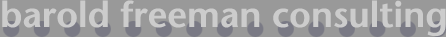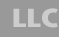### Determining precision with proportions

This form will calculate the precision of any proportion. It is based on formulas from the binomial distribution. Events p and q represent the proportion of results in a dichotomous variable, e.g., heads/tails, yes/no, bush/gore, etc.. Proportions or probabilities must sum to unity (1). Three standard confidence levels are available to choose from.

Select desired confidence level:

Probability of event p:     (e.g., .75)

Probability of event q:     (e.g., .25)

email bfc | phone 608.220.1639